# Matrix of transition probabilities

(diff) ← Older revision | Latest revision (diff) | Newer revision → (diff)

The matrixof transition probabilities in timefor a homogeneous Markov chain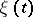with at most a countable set of states:The matrices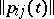of a Markov chain with discrete time or a regular Markov chain with continuous time satisfy the following conditions for anyand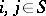: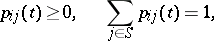i.e. they are stochastic matrices (cf. Stochastic matrix), while for irregular chains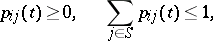such matrices are called sub-stochastic.

By virtue of the basic (Chapman–Kolmogorov) property of a homogeneous Markov chain,the family of matrices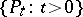forms a multiplicative semi-group; if the time is discrete, this semi-group is uniquely determined by.